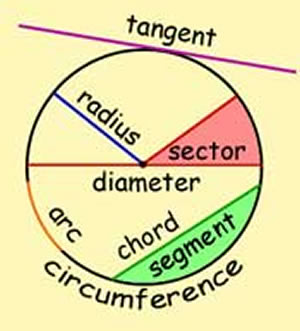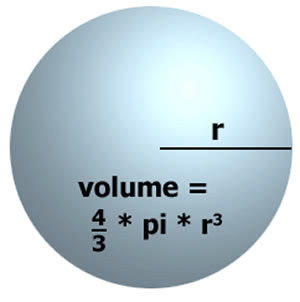Difference between Circle and Sphere

Key difference: Both, circles and spheres are circular objects.  A circle is a two-dimensional figure, while a sphere is a three-dimensional figure.A circle is a round plane figure whose boundary (the circumference) consists of points equidistant from a fixed point (the centre). The circle exists in two-dimension and in a plane. It is a simple shape of Euclidean geometry, in which, a set of all points in a plane are at a fixed given distance from a given fixed point, known as the centre. A circle is a simple closed curve which divides the plane into two regions: an interior and an exterior. Technically, it is known as a disk. It is a curve, which keeps a fixed distance, when traced out by the center point. Its study and development is applicable in the fields of mathematics, geometry, astronomy and calculus. The real world examples are wheels, a dinner plate, the surface of a coin, etc.

The terminology of a circle includes the following definitions:

• Centre: the point equidistant from the points on the circle.
• Radius: a line segment joining the centre of the circle to any point on the circle itself; or the length of such a segment, which is half a diameter.
• Diameter: a line segment whose endpoints lie on the circle and which passes through the centre; or the length of such a line segment, which is the largest distance between any two points on the circle. It is a special case of a chord, namely the longest chord, and it is twice the radius.
• Circumference: the length of one circuit along the circle.
• Chord: a line segment whose endpoints lie on the circle.
• Tangent: a coplanar straight line that touches the circle at a single point.
• Arc: any connected part of the circle.A sphere is a round solid figure, by its surface, with every point on its surface equidistant from its centre. It is a three dimensional figure, which consists of volume. It resembles a shape of a ball. This distance r is the radius of the sphere, and the middle point is the center of the sphere. The maximum straight distance through the sphere passes through the center and is thus twice the radius; it is the diameter. Any plane that includes the center of a sphere divides it into two equal "hemispheres". Archimedes derived the formula of a sphere. It is also defined as the surface formed by rotating a circle about any diameter. Any cross section through a sphere is a circle. Likewise in a circle, all the points in the sphere are at a fixed distance from its center. The examples of sphere in nature are bubbles, planets and small water drops.

Some basic properties of sphere are:

• The points on the sphere are all the same distance from a fixed point. Also, the ratio of the distance of its points from two fixed points is constant.
• The contours and plane sections of the sphere are circles.
• The sphere has constant width and constant girth.
• The sphere does not have a surface of centers.
• The sphere has the greatest volume and the smallest surface area.
• The sphere has constant mean curvature.

Comparison between Circle and Sphere:

 Circle Sphere Definitions A circle is a shape with all points at the same distance from its center. A sphere is a solid figure that is completely round, with every point on its surface at an equal distance from the centre What it is A circle is a figure. A sphere is a object Dimensions It is 2–dimensional figure. It is 3–dimensional figure. Components Here, only surface area can be calculated. Here along with surface area, volume can also be calculated. Important formulas Area = π r² Area = 4 π r²  Volume = 4/3 π r³ Common Examples Bangles and tires. Tennis balls and planets.

Image Courtesy: basic-concept-in-geometry.blogspot.com, calculateme.com